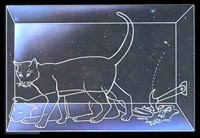The measurement problemSchrödingers's cat (1935-?)
home

## The measurement problem in quantum mechanics

### Definition

Quantum mechanics describes a system by a "wavefunction", or more general by a "state vector". If a system has a specific "property", say a definite position or momentum, it is said to be in an "eigenstate" (more precisely: in an eigenstate with respect to the "operator" which represents the particular quantity. The corresponding "eigenvalue" is the value of the specific quantity). To "be in an eigenstate" is meant in contrast to "being in a superposition" of such eigenstates. The Schrödinger equation dictates the time-evolution of the state-vector, i.e. how it evolves in time given the specific forces or potentials which characterize the system. The specific type of time-evolution is called "unitary", however, for the moment it is enough to know that the evolution is such that given a superposition of different eigenstates in the begining the final state remains in general a superposition. Born's rule states, that the square of the coefficients of the superposition correspond to the probability to obtain the corresponding eigenvalue as result of a measurement.

Now we have everything in place to state the "measurement problem" (MP). It is based on the following dilema: also a "measurement" of some property is a specific form of interaction (namely an interaction between the "system" and the "apparatus" which should be ruled by quantum mechanics.), i.e. turns superpositions into superpositions. However, given that every measurement has a definite outcome it turns the system apparently into an eigenstate!

This dilemma is sometimes expressed by saying that the act of "measurement" forces the state to "collapse" or to "jump" into an eigenstate. However, how does this "non-unitary" evolution relate to the Schrödinger equation? The "collapse" or "reduction" of the state vector is as such no solution to the above dilemma but only an other way to name it.

It is widely held that the measurement problem is the central problem of quantum mechanics. As far as I can see, the different interpretations of quantum mechanics can be classified according to their way how they tackle this problem. In principle there seem to be three main strategies available: (i) one can introduce additional structure (some times called "hidden variables") to distinguish the part of the superposition which corresponds to the measurement outcome. (ii) Some have tried to alter the Schrödinger equation to account for a reduction of the wavefunction as a physical process. (iii) One may view (i.e. define or interpret) the wavefunction as something "non-physical" or "not-only-physical" such that the "collapse" reflects something like principle limitations in the way in which we have access to the physical. The last statement is intentionally vague to cover all sorts of Copenhagen variants or the like. See here for some reflections and resources on the different interpretations of quantum mechanics.

### History of the measurement problem

The standard reference for the collapse of the wavefunction as a special type of time-evolution is von Neumann's "Mathematische Grundlagen der Quantenmechanik" (Springer, Berlin 1932) and here especially Chapter VI. Here the "collapse" is called "projection" (i.e. the superposition state is projected onto the subspace which corresponds to the outcome). Note, that according to common scholarship, Niels Bohr never referred to this concept!

The most often quoted reference for the recognition that the measurement in quantum mechanics is indeed problematic is surly the 3-part paper by Schrödinger from 1935 (1.part, 2.part and 3.part). There he introduced (among other - even more important - things) his famous cat which acts as a measurement device for a quantum process (the decay of a radioactive substance). These papers were a reaction to the famous EPR work. Also in direct response to EPR Henry Margenau pointed to the "projection postulate" as the weak spot in the foundation of quantum mechanics (Henry Margenau, 1936).

A broader interest in the foundational questions seemed to reappear only at the begining of the 60s. A classic paper from this time is Wigner's "The Problem of Measurement" from 1963. Another piece from that time is Henry Margenau (1961).

A good deal of the literature on the measurement problem is devoted to the explication that there really is such a problem. For example Fine makes this point in "Insolubility of the Quantum Measurement Problem" (Phys.Rev.D 2, (1970) 2783-2787). An other example is Ballentine (1988) who uses the measurement problem to advertise his "statistical interpretation" of quantum mechanics. Similarly do Bassi and Ghirardi provide "A General Argument Against the Universal Validity of the Superposition Principle" quant-ph/0009020 (and Phys.Lett. A 275 (2000) 373). Gebhard Grübl's "The quantum measurement problem enhanced" quant-ph/0202101 (and Phys. Lett. A 316 (2003) 153-158.) is an other example for this strategy.

At the same time other argue that the measurement problem is not "real" or has been solved already. See for example "Is there a quantum measurement problem?" by Moldauer (1972).

Rather often one can hear that "decoherence" has solved the measurement problem. However, not even the most important exponents of this line of research make this claim (see for example Zeh's "Roots and Fruits of Decoherence" quant-ph/0512078, p.9). An other critical examination of this claim was written by Adler: "Why Decoherence has not Solved the Measurement Problem: A Response to P. W. Anderson".

This piece by Tim Maudlin from 1995 is a modern and very systematic formulation of the measurement problem: Maudlin, T. (1995), "Three Measurement Problems", Topoi 14, 7-15.